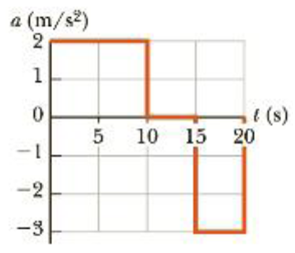Chapter 2, Problem 20P

Chapter
Section
Textbook Problem

A particle starts from rest and accelerates as shown in Figure P2.20. Determine (a) the particle’s speed at t = 10.0 s and at t = 20.0 s, and (b) the distance traveled in the first 20.0 s.Figure P2.20

(a)

To determine
The speed of particle at 10.0s and 20.0s .

Explanation

Given Info: The acceleration till 10.0s is 2.00m/s2 , the initial velocity is 0 , the time is 10.0s , the acceleration in the interval 10.0s to 15.0s is 0 , and the length of the interval is 5.00s .

Explanation:

The formula used to calculate the velocity is,

v=v0+at

• v is the final velocity
• v0 is the initial velocity
• a is the acceleration of the particle
• t is the time

Substitute 0 for v0 , 2.00m/s2 for a and 10.0s for t to find v .

v=0+(2m/s2)(10.0s)=20.0m/s

Thus, the speed of particle at 10.0s is 20.0m/s .

The formula used to calculate the velocity is,

v15=v+a1Δt

• v is the velocity at 10.0s
• v15 is the velocity at 15.0s
• a1 is the acceleration of the particle
• Δt is the time

Substitute 20.0m/s for v , 0 for a1 and 5.00s for Δt to find v15 .

v15=20.0m/s+(0)(5

(b)

To determine
The distance travelled in first 20.0s .

Still sussing out bartleby?

Check out a sample textbook solution.

See a sample solution

The Solution to Your Study Problems

Bartleby provides explanations to thousands of textbook problems written by our experts, many with advanced degrees!

Get Started